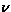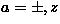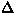Next: A.3 Relaxation function in randomness Up: A Appendix Previous: A.1 Extended Lieb-Shultz-Mattis Theorem

## A.2 The 10/3 effect [#!AndersonRMP53!#,#!KuboTomitaJPSJ54!#]

This section considers nuclear spin relaxation in a paramagnetic localized spin system, with the interaction between the moments described by the dipolar fields.

We assume the Hamiltonian of the spin system to be:

where, H0 is the external magnetic field applied in the z-direction,is the spin of the i-th localized electron and Dij represents the tensor for the dipolar interaction, namely,

where,is the distance between the i-th and the j-th electrons andis the unit vector parallel to the distance.

It is easily shown that the fluctuation rate () of the electron moments doesn't depend on the external field; if one writes the Hamiltonian (eq.68) in the rotating reference frame (RRF) for the electrons, one can eliminate the external field, while the dipolar interaction is expressed as:

whereis the first term of the Hamiltonian (eq.68) andthe RRF representation of the spin. This result indicates that the dipolar interaction between the electrons (namely the associated fluctuation rate) doesn't change in RRF, because the interacting two spinsandhave the same gyromagnetic ratio, and conduct coherent precession in the external field H0.

To the probe nuclear spin, the Larmor precession of the electron moment in a reasonably large external field H0 is so fast that its xy components becomes invisible (Fig.64). In this limit, the probe spin detects only the z-component of the electron moments, which changes its length with the dipolar fluctuation rate (). In this situation, the T1 relaxation rate of the probe spin may be reduced byfrom that in the zero-field case, because the average size of the z-component squared is 1/3 of the full spin:. As shown below, the precise factor is 3/10.Generally, the T1 relaxation rate of a probe nuclear spin is expressed as :

where,is the Larmor precession frequency of the nuclear spin (muon),(a=x,y) is the x,y-component of the fluctuating local fields at the probe spin site and. The bracketdenotes the ensemble average. If the muon-electron interaction is the dipolar fields, the local field at the muon site becomes:

whereis the distance between the muon and the j-th electron andis the unit vector parallel to the distance.

To write, it is convenient to define, where,(a=x,y) is the x,y-component of the unit vector. The local fields are expressed as:

Using the rotating reference frame (RRF) for the electrons, the electron spin correlations <Saj(0)Sbk(t)> () are expressed as:

whereis the electron Larmor frequency, Saj;R(t) () is the electron spins expressed in the RRF. Assuming a Markoffian fluctuation processes, decay of the spin correlation is expressed as

whereis the dipolar fluctuation rate, and. In paramagnetic state, there is no correlation between the j-th and the k-th spin, yielding the t=0 correlation functions as:

Using the above presented expressions, the field correlation at the probe spin site becomes:

In the same manner,is calculated, yielding the T1 relaxation rate as:

In an isotropic sample, the coefficients are replaced by the angular averages:

Introducing the dipolar field width () defined in eq.45, the T1 relaxation yields:

Namely, if the Larmor precession of the electron moments are fast, the local field width at the muon site is effectively reduced to.Next: A.3 Relaxation function in randomness Up: A Appendix Previous: A.1 Extended Lieb-Shultz-Mattis Theorem